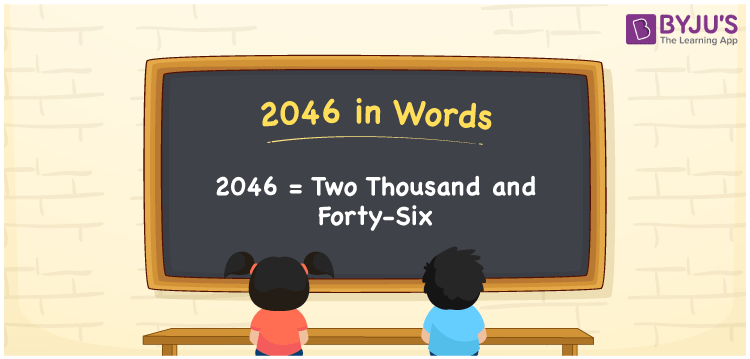# 2046 in Words

2046 in words is written as Two thousand forty-six. In both the International System of Numerals and the Indian System of Numerals, 2046 is written as Two thousand forty-six. The number 2046 is a Cardinal Number as it could represent some quantity. For example, “the speakers cost 2046 rupees”.

 2046 in Words Two thousand forty-six Two thousand forty-six in Number 2046

## 2046 in English Words

2046 in English words is read as “Two thousand forty-six”.## How to Write 2046 in Words?

To write 2046 in words, we shall use the place value chart. In the place value chart, put 2 in the thousands, 0 in the hundreds, 4 in the tens, and 6 in the ones, respectively. Let us make a place value chart to write the number 2046 in words.

 Thousands Hundreds Tens Ones 2 0 4 6

Thus, we can write the expanded form as

2 × Thousand + 0 × Hundred + 4 × Ten + 6 × One

= 2 × 1000 + 0 × 100 + 4 × 10 + 6 × 1

= 2000 + 0 + 40 + 6

= 2046

= Two thousand forty-six.

2046 is a natural number, the successor of 2045 and the predecessor of 2047.

2046 in words – Two thousand forty-six

• Is 2046 an odd number? – No
• Is 2046 an even number? – Yes
• Is 2046 a perfect square number? – No
• Is 2046 a perfect cube number? – No
• Is 2046 a prime number? – No
• Is 2046 a composite number? – Yes

## Frequently Asked Questions on 2046 in Words

Q1

### How to write 2046 in words?

2046 in words is written as Two thousand forty-six.
Q2

### How to write 2046 in the International and Indian System of Numerals?

In both, the system of numerals, 2046 in words, is written as Two thousand forty-six.
Q3

### How to write 2046 in a place value chart?

In the place value chart, write 2 in the thousands, 0 in the hundreds, 4 in the tens, and 6 in the ones, respectively.